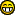News: Visit the official fractalforums.com Youtube ChannelWelcome, Guest. Please login or register. June 14, 2021, 04:18:13 AM 1 Hour 1 Day 1 Week 1 Month Forever Login with username, password and session length

## The All New FractalForums is now in Public Beta Testing! Visit FractalForums.org and check it out!

 Pages:    Go DownAuthor Topic: Bounding circle of julia sets  (Read 3215 times) Description: Who said it's radius is two? 0 Members and 1 Guest are viewing this topic.
knighty
Fractal IambusPosts: 819« on: December 11, 2010, 08:47:52 PM »

Hello,
This is quite simple and you may already know it but I couldn't find any reference in the net:
The question is what is the radius of the smallest circle bounding a given julia set? I found a formula that gives that radius when c is real and is <= 0. For other c's it gives very good bounding circle. an interresting fact about the bounding circles found with this formula is that when their radius is used as the bailout, the leminscates are tangent.
The radius "r" is the positive solution of the equation: r^p-r-|c|=0
Where p is the power of the julia set (in z<-z^p+c).

Here are two evaldraw srcipts for illustration:
Code:
static n=2;
(x,y) {
static cx=-1,cy=0.5;
static bo=2;
static frm=-1;
if(numframes>frm){
frm=numframes;
cx=(mousx-0.5*xres)*0.005;
cy=(0.5*yres-mousy)*0.005;
bo=newton(1,sqrt(cx*cx+cy*cy))^2;
}
1-julia(x,y,cx,cy,32,bo)
}
julia(x,y,cx,cy,it,bo){
r2=x*x+y*y;
for(i=0;i<it && r2<bo;i++){
r=sqrt(r2);
t=atan2(y,x);
r=r^n;t=n*t;
x=r*cos(t)+cx;
y=r*sin(t)+cy;
/*x1=x*x-y*y+cx;
y=2*x*y+cy;
x=x1;*/
r2=x*x+y*y;
}
i/it
}
newton(x,c){//Solves for the radius
i=0;
do{
x1=x-(x^n-x-c)/(n*x^(n-1)-1);
if(abs(x-x1)/x<2^-53) break;
x=x1;
i++;
}while(i<5);
x
}

Code:
static n=2;
(x,y) {
cx=x;cy=y;
bo=newton(1,sqrt(cx*cx+cy*cy))^2;
1-julia(x,y,cx,cy,32,bo)//in fact this is the mandelbrot set :) replace "bo" by 2 and see the difference
}
julia(x,y,cx,cy,it,bo){
r2=x*x+y*y;
for(i=0;i<it && r2<bo;i++){
r=sqrt(r2);
t=atan2(y,x);
r=r^n;t=n*t;
x=r*cos(t)+cx;
y=r*sin(t)+cy;
/*x1=x*x-y*y+cx;
y=2*x*y+cy;
x=x1;*/
r2=x*x+y*y;
}
i/it
}
newton(x,c){
i=0;
do{
x1=x-(x^n-x-c)/(n*x^(n-1)-1);
if(abs(x-x1)/x<2^-53) break;
x=x1;
i++;
}while(i<5);
x
}

I haven't tried it yet with quaternion julia and julia bulbs but I think it should work with them.Logged
Adam Majewski
Fractal LoverPosts: 221« Reply #1 on: September 07, 2011, 03:42:44 PM »

Hi,
It is described in paper :
Julia Sets of Complex Polynomials and Their Implementation on the Computer by Christoph Martin Stroh

HTH
AdaLogged
Fractal Ken
Fractal LoverPosts: 246Proud to be 2D« Reply #2 on: September 07, 2011, 06:23:04 PM »

It is described in paper :
Julia Sets of Complex Polynomials and Their Implementation on the Computer by Christoph Martin Stroh

Here's the link.Logged

Fortran will rise again
knighty
Fractal IambusPosts: 819« Reply #3 on: September 07, 2011, 07:57:41 PM »

Thank you very much.
Very interesting document even if it is maybe too technical for my small brain. How did you find it?Logged
Adam Majewski
Fractal LoverPosts: 221« Reply #4 on: September 07, 2011, 11:24:43 PM »

I have found it with google .
Please look also here :
http://en.wikibooks.org/wiki/Fractals/Iterations_in_the_complex_plane/Julia_set

AdamLogged
knighty
Fractal IambusPosts: 819« Reply #5 on: September 08, 2011, 08:44:50 PM »

Well... I'll have to improve my "googling" skills.
So you are the author of that wikibook! Excellent! Thank you for citing this thread. Just one note: the formula I've found is exactly the same as Stroh's. Moreover he had mathematically justified and generalized it.
BTW welcome to FF.Logged
Adam Majewski
Fractal LoverPosts: 221« Reply #6 on: September 11, 2011, 01:05:05 PM »

>So you are the author of that wikibook!
I started only some pages not the whole book.
Note that I use code made by other people so there are more authors.

>Excellent! Thank you for citing this thread. Just one note: the formula I've found is exactly the same as Stroh's
You can edit and change it or add new things.
There are many (new or old but hard to implement) algorithms even in case of http://en.wikipedia.org/wiki/Complex_quadratic_polynomial
Help is wellcomeLogged
knighty
Fractal IambusPosts: 819« Reply #7 on: September 13, 2011, 07:54:28 PM »

Thank you for the invitation.Logged
 Pages:    Go Down
Jump to:

 Related Topics Subject Started by Replies Views Last postVirtual investigation to Mandelbrot sets and Julia sets Mandelbrot & Julia Set Jules Ruis 0 2431October 19, 2006, 06:36:54 PM by Jules RuisFun with Dynamic Spirograph: Circle Rolling around Rolling Circle Non-Fractal related Chit-Chat « 1 2 » benice 19 5219February 11, 2012, 01:20:47 AM by SockrateaseLattès Julia sets Mandelbrot & Julia Set s31415 1 1261April 29, 2013, 12:33:52 AM by Pauldelbrotfractal dimension of Julia sets Mandelbrot & Julia Set claude 12 2692July 26, 2016, 03:35:34 AM by valera_rozuvanJulia-sets for continous functions (new) Theories & Research SamTiba 4 252February 05, 2017, 10:20:16 AM by SamTiba

Page created in 0.109 seconds with 27 queries. (Pretty URLs adds 0.006s, 2q)